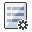The Rocscience International Conference 2021 Proceedings are now available. Read Now

# Overview of a Combinations Analysis

The Combination analysis option in SWedge allows you to enter any number of discrete joint plane orientations, and all possible combinations of two joints which can form a wedge will be analyzed. The wedge with the minimum factor of safety is determined, and results can be plotted on histograms, scatter plots and the Stereonet view.

NOTE: See the Combination Analysis Tutorial for a practical example which illustrates the use of this option.

The general procedure for carrying out a Combinations Analysis with SWedge is as follows.

1. ## Set Analysis Type

Set the Analysis Type to Combinations in the Project Settings dialog.

2. ## Define Joint Properties

In the Input Datadialog, the joint orientation data can be entered directly or imported from a Dips file. You may define a single set of joint data or two sets. If two sets are defined, then the two sets can have different strength properties, and all possible combinations (using one joint from each set), will be analyzed.
3. ## Define Slope Properties

Slope Properties, Tension Crack, and Forces are defined in the same way as for a Deterministic analysis, see the following topics for details.

4. ## Compute

The Combination Analysis is computed when you select OK (or Apply) in the Input Data dialog, or when support is added or modified.

5. ## View Analysis Results

After the analysis is computed, the Sidebar will display a summary of results for the wedge with the minimum safety factor. Results can be plotted on Histogram plots, Scatter plots etc. Data can also be exported for further analysis and processing. A summary of all input and output data, can be found in the Info Viewer.